Solving Inequalities: An Overview (page 1 of 3)

Sections: Linear inequalities, Quadratic inequalities, Other inequalities

Solving linear inequalities is very similar to solving linear equations, except for one small but important detail: you flip the inequality sign whenever you multiply or divide the inequality by a negative. The easiest way to show this is with some examples:

 1)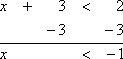Graphically, the solution is: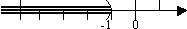The only difference between the linear equation "x + 3 = 2" and this linear inequality is that I have a "less than" sign, instead of an "equals" sign. The solution method is exactly the same: subtract 3 from either side. Note that the solution to a "less than, but not equal to" inequality is graphed with a parentheses (or else an open dot) at the endpoint, indicating that the endpoint is not included within the solution. 2)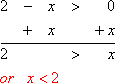Graphically, the solution is: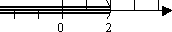The only difference between the linear equation "2 – x = 0" and this linear inequality is the "greater than" sign in place of an "equals" sign. Note that "x" in the solution does not "have" to be on the left. However, it is often easier to picture what the solution means with the variable on the left. Don't be afraid to rearrange things to suit your taste. 3)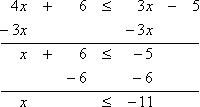Graphically, the solution is: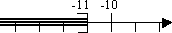The only difference between the linear equation "4x + 6 = 3x – 5" and this inequality is the "less than or equal to" sign in place of a plain "equals" sign. The solution method is exactly the same. Note that the solution to a "less than or equal to" inequality is graphed with a square bracket (or else a closed dot) at the endpoint, indicating that the endpoint is included within the solution. 4)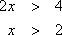Graphically, the solution is: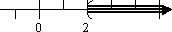The solution method here is to divide both sides by a positive two.     Copyright © Elizabeth Stapel 1999-2011 All Rights Reserved 5)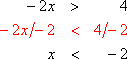Graphically, the solution is: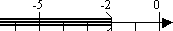This is the special case noted above.  When I divided by the negative two, I had to flip the inequality sign.

The rule for example 5 above often seems unreasonable to students the first time they see it. But think about inequalities with numbers in there, instead of variables. You know that the number four is larger than the number two: 4 > 2. Multiplying through this inequality by –1, we get –4 < –2, which the number line shows is true: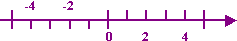If we hadn't flipped the inequality, we would have ended up with "–4 > –2", which clearly isn't true.

Top  |  1 | 2 | 3  |  Return to Index  Next >>

 Cite this article as: Stapel, Elizabeth. "Solving Inequalities: An Overview." Purplemath. Available from     https://www.purplemath.com/modules/ineqsolv.htm. Accessed [Date] [Month] 2016

MathHelp.com Courses